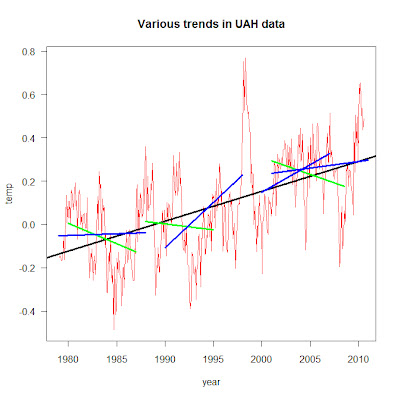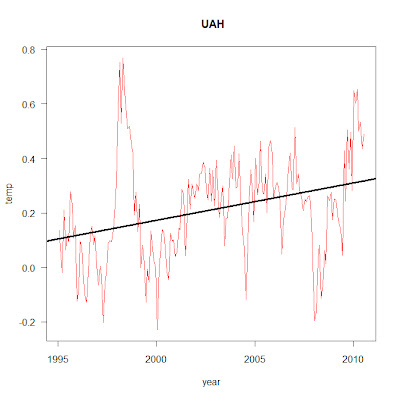Gadget by The Blog Doctor.

## Friday, August 20, 2010

### Global Warming Has Not Stopped

Introduction

It is often claimed by Climate "Skeptics" that Global Warming has stopped. This post will examine that claim.

Trends

The chart below plots the data set developed at the University of Alabama at Huntsville (UAH). I decided to use this data set as it is the one that is most popular with Climate "Skeptics". The data can be accessed here. The blog of one of the leaders of this group can be found here. As always on this blog, click on the graphic to see a larger and clearer version.I produced this chart using the downloaded data in a statistical programming language called R. The script that I wrote can be found in Appendix 1 at the end of this post.

The red line charts the monthly data. The temperatures are quite variable, but just by eyeballing the plot, it is clear that there is a rising trend to the data. The black line is the linear best fit to the data set. According to the UAH data (bottom of page) the trend is 0.138 degrees celsius per decade. Over thirty years that trend will produce a 0.4oC of warming globally, but over shorter periods of time (say ten years or less) 0.138oC can be easily overwhelmed by the much larger year on year temperature changes. Consequently, trends taken over short time periods can show no trend, or a rising trend, or a declining trend, as can be seen clearly in the chart. Over time the more or less random variations cancel out and the real trend appears.

One feature that stands out clearly in the chart is the spectacularly warm year of 1998. This was caused by a very strong El Nino. For a few years, Climate "Skeptics" claimed that Global Warming stopped in 1998. Here is one example of such a claim. It is hardly surprising that the years immediatley following 1998 would be cooler. A problem arose for the "skeptics" when trends from 1998 to 2005, 2006 and 2007 showed an upward trend (click on years to see trend charts). The solution was simple - find a new date where a declining trend could be observed. In order to do this the "skeptics" had to move on four years in the temperature data - to 2002. Here is an article from 2008 that now states that Global Warming stopped not in 1998 but in 2002. The usual problem for the "sketics" is reappearing as the trend from 2002 is starting to appear in the data. See this link for a chart from 2002 to 2010.

It is clear that the only way to sustain an argument that atmospheric temperatures are cooling is by carefully choosing short periods of time to construct trends.

Statistical Significance

Another attempt by "skeptics" to cast doubt on the warming trend is to claim that "there has been no statistically significant warming in the atmospheric temperature record since 1995". By a process of Chinese Whispers this becomes "no significant warming since 1995" to "no warming since 1995" to "the planet is cooling". Here is an example of such an argument.

Before considering the meaning of statistically significant warming it is instrutive to investigate the trend from 1995. When reading articles like the one that I linked to in the previous paragraph, one would could assume that there has not been any trend in atmospheric temperature since 1995, but that view is clearly not true as shown by the chart below:So far in this post I have only discussed one of the temperature data sets. In fact there are five of them - GISS, NOAA, Hadley, RSS as well as UAH. This post discusses the differences and similarities of all five groups. The video below shows trends for all five goups to the current date, from 1995 to 2008. Note that 90% of the trends are increasing. The script that I wrote to calculate the trends in the video is reproduced in Appendix 2.

The concept of statistical significance is quite complex and a proper understanding of it requries considerable study of statistics. Here is a post that discusses the idea of statistical significance, here is a post that discusses the claim that temperature data sets show no statistically significant warming since 1995, and here is a link to a video discussing the same information.

What is clear is that it is very likely that the temperature trends since 1995 are real. Technically it is not quite true to claim that the trends from 1995 are at least 92% probable, but that claim gives a sense of the issue. What is true is that the trends in all five temperature time series are statistically significant from 1994.

Other Indicators that the Planet is Warming

Temperatures in the atmosphere are not the only indicators that the globe is warming. Ten warming indicators are listed below, note that seven of them are not related to atmospheric temperatures.

Each of the ten indicators are changing in the direction that would be expected if the planet were warming.

Indicators that increase in a warming world:

Air temperature over land
Air temperature over sea
Troposphere temperature
Sea surface temperature
Ocean heat content
Humidity
Sea level

Indicators that decrease in a warming world:

Snow coverage
Sea ice extent
Glaciers

For more infirmation and evidence on these indicators see this post.

Conclusion

There are three major reasons that disprove the claims that global temperatures are not increasing:
1. their reliance on short time preiods to produce trends - which is statistically absurd
2. their use of the complex concept of statistical significance to give a false impression to those who don't understand any statistics
3. their reliance on just one of the many indicators of warming temperatures.

Given the weakness of these arguments, it is hardly surprising that the vast majority of people qualified to make a judgement are sure that global temperatures are increasing.

UPDATE: 09/09/2010 While I was writing this post I wondered whether Andrew Bolt (Australia's premier denier) would recognise the current warming reality. Finally he has done so at this post. Interestingly, Bolt doesn't comment on Roy Spencer's graph. He also points to an SBS show with the late Stephen Schneider, who politely explained to a large group of "skeptics" why their objections to AGW did not make sense. In the post Bolt also returned to his tried and true technique of misrepresenting the views of climate scientists, this time Tong Lee Michael McPhaden. See this post for a clearer discussion of the work on ENSO. Lastly Bolt continues to attempt to confuse his readers on Arctic sea ice.

________________________________________________________________________________

Appendix 1

#################### UAH_53 user defined trends #################
## Written by Stephen Spencer, Tarneit, Victoria, Australia
## Date 20/08/2010

## STEP 1: SETUP - Source File
par(las=1)
link <- "C:\\Learn_R\\All_temp_groups.csv" ## STEP 2: READ DATA my_data <- read.table(link, sep = ",", dec=".", skip = 0, row.names = NULL, header = T, colClasses = rep("numeric", 2), na.strings = c("", "*", "-", -99.99,99.9, 999.9), col.names = c("yr","GISS","RSS","NOAA","UAH","HAD","UAH_53")) ## STEP 3: MANIPULATE DATA Title <- "Various trends in UAH data" #user defined function to calculate trend data #for user entered years calc_trend = function (start_date, end_date){ newdata <-(subset(my_data, yr>=(start_date +.042) & yr<= (end_date + .985)))
first_lm = (lm(UAH_53 ~ yr, data = newdata))
a <- coef(first_lm)
b <- coef(first_lm)
x_vals <<- c(start_date, end_date)
y_vals <<- c(a+b*(start_date +.042), a + b*(end_date + .985))}

## STEP 4 PLOT DATA AND TRENDS

# PLOT UAH_53 DATA FROM 1979 AND ADD TREND LINE
plot(UAH_53 ~ yr, data = my_data, type="l", col = "red", xlab = "year", ylab = "temp", main = Title)
full_lm = lm(UAH_53 ~ yr, data = my_data)
abline(full_lm, lwd=3)

#CALCULATE TREND LINES FOR SHORT TIME PERIODS

#Calculate and draw trend from 1980 to 1987
calc_trend (1980, 1987)
lines(x_vals, y_vals, col = "green", lwd=3)

#Calculate and draw trend from 1988 to 1995
calc_trend (1988, 1995)
lines(x_vals, y_vals, col = "green", lwd=3)

#Calculate and draw trend from 2001 to 2008
calc_trend (2001, 2008.5)
lines(x_vals, y_vals, col = "green", lwd=3)

#Calculate and draw trend from 1979 to 1988
calc_trend (1979, 1988)
lines(x_vals, y_vals, col = "blue",lwd=3)

#Calculate and draw trend from 1990 to 1998
calc_trend (1990, 1998)
lines(x_vals, y_vals, col = "blue", lwd=3)

#Calculate and draw trend from 2000 to 2007
calc_trend (2000, 2007)
lines(x_vals, y_vals, col = "blue", lwd=3)

#Calculate and draw trend from 2000 to 2010
calc_trend (2001, 2010.99)
lines(x_vals, y_vals, col = "blue", lwd=3)

Appendix 2

########## All data and trend analysis from userdefined date ######

## STEP 1: SETUP - Source File Link
par(las=1)

## STEP 2: READ DATA
sep = ",", dec=".", skip = 0,
row.names = NULL, header = T,
colClasses = rep("numeric", 2),
na.strings = c("", "*", "-", -99.99,99.9, 999.9),

## STEP 3: MANIPULATE DATA
## Change the year in the next line to the year that
# you want to plot the trends from, not before 1979!
yearfrom = 1995
# Create title for chart
Title <- c("All data trends from", yearfrom)
# Create a new data set from the selected year
newdata <-(subset(my_data, yr>=yearfrom))

## STEP 4: CREATE CHART AND DRAW TREND LINES
# Display only trend lines
# Data has to be plotted so that abline operates
# So plot data in white
plot(GISS ~ yr, data = newdata, type="l", col = "white", xlab = "Year", ylab = "Temperature", main = Title)
# Draw trend lines
abline(lm(GISS ~ yr, data = newdata), col = "blue", lwd=3)
abline(lm(RSS ~ yr, data = newdata), col = "red", lwd=3)
abline(lm(NOAA ~ yr, data = newdata), col = "green", lwd=3)
abline(lm(HAD ~ yr, data = newdata), col = "purple", lwd=3)
abline(lm(UAH_53 ~ yr, data = newdata), col = "black", lwd=3)

## Insert text annotations
# Calculate x position for text command
x_pos <- yearfrom + 1
# Display names of data sets in appropriate colours
text(x_pos,0.8, "GISS", col="blue", cex=1.5, ps = 20)
text(x_pos,0.75, "NOAA", col="green", cex=1.5, ps = 20)
text(x_pos,0.7, "HADLEY", col="purple", cex=1.5, ps = 20)
text(x_pos,0.65, "RSS", col="red", cex=1.5, ps = 20)
text(x_pos,0.6, "UAH", col="black", cex=1.5, ps = 20)